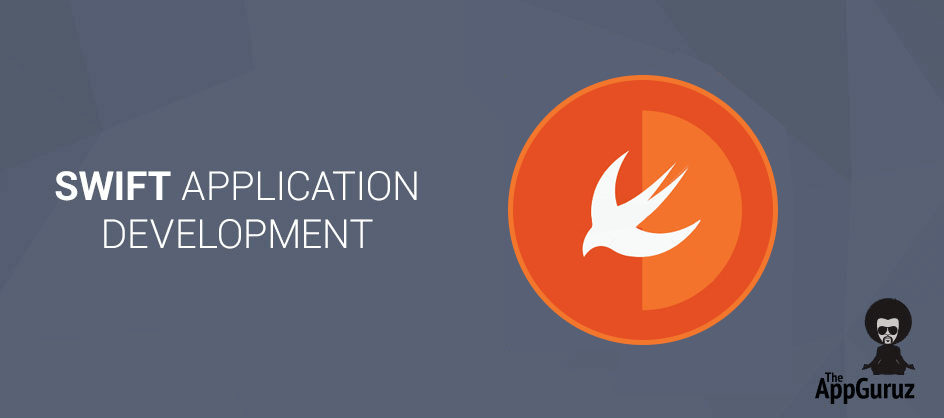## Objective

This tutorial teaches you how to write code in swift to make iPhone app quickly.

Swift is a new programming language for iOS and OSX project. Swift comes as the result of research on many programming language. The syntax of swift looks like as C and Objective C language.

This tutorial gives information about variable, Control flow, Functions and Closures, Objects and Classes etc. For this Swift tutorial you required Xcode 6-Beta.

## Step 1Variable Declaration

``````var mutableDoubleVar: Double = 1.0
let constantDoubleVar: Double = 1.0``````

Here we are using var to declare variable and let to declare constant. We can assign the value of constant single but can use it in many places. Also we can declare variable with other variable type same like this. In Swift language; there is no need to specify the type of variable always. By assigning the value to variable or constant compiler identify the type of variable. In below example intVar is of type integer because it contains Integer value and doubleVar is of type Double as it contains Double value.

``````let intVar = 70
let doubleVar = 70.0``````

Also we can include other variables in string using backslash and round parenthesis (“Pen Value: \(pen)”) as shown in below example.

``````let pen = 3
let pencil = 5
let string1 = “I have \(pen) pens”
let string2 = “I have \(pencil) pencils”``````

## Step 2Other Variable Types

``````Int  - 1, 100, 5000
Float, Double  - 1.2, 10.0, 500.5
Bool – true, false
String – “StringValue”
ClassName – UIView, UILabel etc.``````

## Step 3Control Flow

In Swift for conditionals use If and Switch and for loops use for-in, for, while and do-while. Around the condition and loops variables parenthesis are optional but around the body curly braces are required. In If statement; the conditional statement has to use only Boolean expression.

``````let studentMark = [75, 85, 90, 95, 70, 80]
for mark in studentMark {
if mark > 80 {
} else {
}
}``````

## Step 4Optional Binding

In swift one feature is there to assign optional value to a variable or constant. To declare variable as a optional have to write question mark(?) after the type of variable. This optional variable or constant contain either value or nil value.

``````var subject = [“Maths”, “Physics”, “Chemistry”, “English”]
func findSubject (data:String[], searchItem:String)-> Int? {
var index
for subject in data {
if subject == searchItem {
returnindex
}
index++
}
return nil
}``````

## Step 5Functions And Closures

Functions are block of code which is written to perform some specific task. We can give any name to function and can use that name to call the function to perform that specific task. To declare a function used func as shown in below example. Also we can pass argument in function in parenthesis and to return variable declare argument after -> which separate the function arguments and return arguments.

``````var subject = [“Maths”, “Physics”, “Chemistry”, “English”]
func findSubject (data:String[], searchItem:String)-> Int {
var index: Int = 0
for subject in data {
if subject == searchItem {
return index
}
index++
}
return Index
}``````

Block of functionality which we can passed around and used in our code also it can capture and store references to any constants and variables in the definded context known as Closures.

``````{ (string1: String, string2: String) -> Bool in
string1 > string2
}``````

## Step 6Tuples

Tuples is a group of multiple in a single value and can declare any type of variable in that no need to declare variable of same type.

### 6.1Unnamed Tuples

Below example shows unnamed tuple means no need to specify name of variable and can access that data using index as shown in example.

``````let amountAndPercent: (Double, Double) = (200, 10)
amountAndPercent.0
amountAndPercent.1``````

### 6.2Named Tuples

Below example shows Named tuple means in that have to specify name of variable and can access that data using that name as shown in example.

``````let amountAndPercent = (amount: 200, percent:10)
amountAndPercent.amount
amountAndPercent.Percent``````

### 6.3Returning Tuples

Below example shows Returning tuple means in can return tuple in function to return multiple value as shown in example.

``````func caculateTotalAndPercent (data:Int[]) -> (amount:Int, percentage:Int) {
let totalAmt: Int = 0
let percentAmt: Int = 10
for amountData in data {
totalAmt  +=amountData
}
return (totalAmount, percentAmt)
}``````

## Step 7Objects And Classes

To create a declare class use class before the name of class. In class can declare property is same like variable and constant declaration. To create an instance of class have to use parenthesis after class name and can use property of class with dot syntax as shown in below example.

``````class Mark {
var totalMark = 0
func countTotalMark(mark:Int[]) {
for markData in mark {
totalMark += markData
}
}
}

var mark = Mark()
mark.countTotalMark
println(“Total Mark : \(mark.totalMark)”)``````

I hope you found this blog helpful while Developing Application in Swit. Let me know if you have any questions or concerns regarding Swift App Development, please put a comment here and we will get back to you ASAP.

Got an Idea of iPhone App Development? What are you still waiting for? Contact us now and see the Idea live soon. Our company has been named as one of the best iPhone App Development Company in India.Being an iOS developer with experience of 3 years feels pretty awesome because much more than just playing around with code, software developers are the brains behind the design, installation, testing and maintenance of software systems.